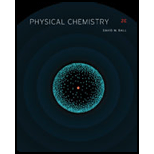# Interstellar space can be considered as having 10 atoms of hydrogen per cubic centimeter and an average temperature (far away from stars!) of 2.7 K . Determine (a) the pressure of hydrogen in interstellar space and (b) the average speed of the hydrogen atoms. Compare these answers with values under normal Earth conditions.### Physical Chemistry

2nd Edition
Ball + 3 others
ISBN: 9781133958437

#### Solutions

Chapter
Section### Physical Chemistry

2nd Edition
Ball + 3 others
ISBN: 9781133958437
Chapter 19, Problem 19.12E
Textbook Problem
20 views

## Interstellar space can be considered as having 10   atoms of hydrogen per cubic centimeter and an average temperature (far away from stars!) of 2.7   K . Determine (a) the pressure of hydrogen in interstellar space and (b) the average speed of the hydrogen atoms. Compare these answers with values under normal Earth conditions.

Interpretation Introduction

(a)

Interpretation:

The pressure of hydrogen in interstellar space is to be calculated.

Concept introduction:

The pressure of a gas is calculated by the formula,

p=2NEavg3V

Where,

N is the number of atoms.

V is the volume.

Eavg is the average energy of the atoms.

### Explanation of Solution

The pressure of a gas is calculated by the formula,

p=2NEavg3V … (1)

Where,

N is the number of atoms.

V is the volume.

Eavg is the average energy of the atoms.

The average energy of the atoms is calculated by the formula,

Eavg=3nRT2N … (2)

Where,

n is the number of moles.

R is the universal gas constant.

T is the temperature.

The number of moles is calculated by the formula,

The number of atoms present in 1cm3 is 10.

The number of atoms present in 1mol is 6.02×1023.

Substitute the number of atoms present in 1cm3 and number of atoms present in 1mol in the above formula.

n=106.02×1023=1.66×1023mol

The value of universal gas constant is 8.205×105m3atmK1mol1.

Substitute the value of number of moles, universal gas constant, and temperature in equation (2).

Eavg=3×1

Interpretation Introduction

(b)

Interpretation:

The average speed of hydrogen atoms is to be calculated.

Concept introduction:

A gas is made up of atoms or molecules that move with very high speeds. The kinetic energy of gases is very high. Every molecule or atom present in a gas can have a different energy. Therefore, root mean square speed, most probable velocity, and mean velocity are calculated for a gas.

### Still sussing out bartleby?

Check out a sample textbook solution.

See a sample solution

#### The Solution to Your Study Problems

Bartleby provides explanations to thousands of textbook problems written by our experts, many with advanced degrees!

Get Started

Find more solutions based on key concepts
What is the structural difference between a lecithin and a cephalin?

Chemistry for Today: General, Organic, and Biochemistry

What does the ground state of an atom represent?

Introductory Chemistry: A Foundation

What is population genetics?

Human Heredity: Principles and Issues (MindTap Course List)

If two stars differ by 8.6 magnitudes, what is their flux ration?

Horizons: Exploring the Universe (MindTap Course List)

Nutrients and drugs can interact before ingestion or absorption. within the body tissues. at the level of gene ...

Nutrition: Concepts and Controversies - Standalone book (MindTap Course List)

What types of symbiosis exist?

Oceanography: An Invitation To Marine Science, Loose-leaf Versin

Describe the physical significance of the Poynting vector.

Physics for Scientists and Engineers, Technology Update (No access codes included)